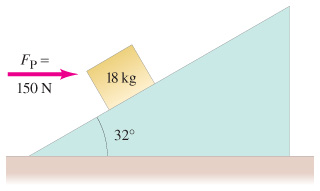# Problem: How much work is done by the horizontal force FP = 150 N on the 18-kg block of the figure when the force pushes the block 6.0 m up along the 32 frictionless incline?How much work is done by the gravitational force on the block during this displacement?How much work is done by the normal force?What is the speed of the block (assume that it is zero initially) after this d is placement? [Hint: Work-energy involves net work done.]

⚠️Our tutors found the solution shown to be helpful for the problem you're searching for. We don't have the exact solution yet.

###### Problem Details

How much work is done by the horizontal force FP = 150 N on the 18-kg block of the figurewhen the force pushes the block 6.0 m up along the 32 frictionless incline?

How much work is done by the gravitational force on the block during this displacement?

How much work is done by the normal force?

What is the speed of the block (assume that it is zero initially) after this d is placement? [Hint: Work-energy involves net work done.]